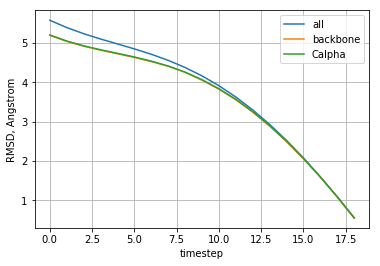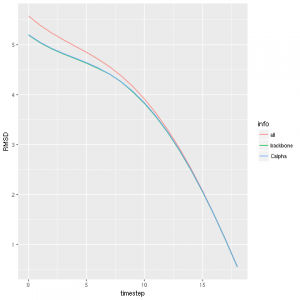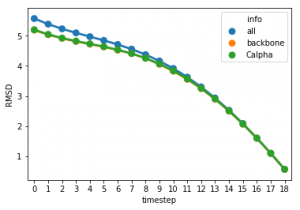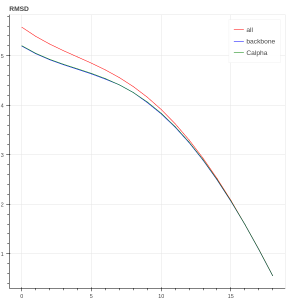# Visualization and exploration of data

### Visualization and exploration of data

There exists a lot of different tools for visualization and exploration of data in Python.

#### Content:

For the purposes of this tutorial, we will compute the root-mean-square deviation of atomic positions (RMSD) of a molecule along a conformation path compared to the goal molecule conformation. We assume that each of the conformations is being stored as a separate pdb-file and these files are loaded into SAMSON according to the conformation path (the first molecule is the starting conformation and the last molecule is the goal). The result will be stored in a pandas data frame.

You can find the code in SAMSON Python Scripting samples on github.

The RMSD is computed using the next script.

```from math import sqrt import pandas as pd   def compute_rmsd(mol1_indexer, mol2_indexer): ''' Computes RMSD between two conformations of a molecule NB: this is not an optimized implementation, it is only for the purpose of this tutorial ''' if mol1_indexer.size != mol2_indexer.size: # check if indexers are of the same size print('molecules are not the same') return -1   rmsd = 0.0; for i in range(mol1_indexer.size): # go through all atoms in the indexer if mol1_indexer[i].elementType != mol2_indexer[i].elementType: print('atoms are not the same') return -1 pos1 = mol1_indexer[i].getPosition() # get position of an atom from an indexer pos2 = mol2_indexer[i].getPosition() distance = pos1 - pos2 # compute distance between atoms rmsd += distance.norm2().angstrom # accumulate squared norm for distances [in angstrom]   rmsd = sqrt(rmsd / mol1_indexer.size) # take a square root of the sum of squared distances divided by the number of atoms return rmsd   def compute_rmsd_2mol(mol1, mol2): ''' Computes several RMSD between two conformations: 1) between all atoms; 2) between backbone atoms; 3) between alpha-Carbon atoms ''' mol1_all_indexer = mol1.getNodes('n.t a') # get all atoms mol2_all_indexer = mol2.getNodes('n.t a') mol1_backbone_indexer = mol1.getNodes('n.t a in n.t bb') # get all atoms in the backbone mol2_backbone_indexer = mol2.getNodes('n.t a in n.t bb') mol1_CA_indexer = mol1.getNodes('"CA" in n.t bb') # get all alpha Carbons in the backbone mol2_CA_indexer = mol2.getNodes('"CA" in n.t bb')   rmsd_all = compute_rmsd(mol1_all_indexer, mol2_all_indexer) rmsd_backbone = compute_rmsd(mol1_backbone_indexer, mol2_backbone_indexer) rmsd_CA = compute_rmsd(mol1_CA_indexer, mol2_CA_indexer)   if (rmsd_all != -1): print("RMSD (all atoms): %e Angstrom" % rmsd_all) if (rmsd_backbone != -1): print("RMSD (backbone) : %e Angstrom" % rmsd_backbone) if (rmsd_CA != -1): print("RMSD (C_alpha) : %e Angstrom" % rmsd_CA)   return [rmsd_all, rmsd_backbone, rmsd_CA], ['all', 'backbone', 'Calpha'] # return list of different RMSD     indexer = SAMSON.getNodes('n.t m') # get all molecules if indexer.size < 2: print("less than two molecules are present in the data graph") else: # we assume here that we need to compute RMSD between the first two molecules goal = indexer[-1] # get the goal molecule [assume the last molecule to be the goal one] indexer.removeNode(goal) # remove the target node from the indexer df = pd.DataFrame() # create pandas data frame for t in range(indexer.size): mol = indexer[t] rmsd, rmsd_info = compute_rmsd_2mol(goal, mol) # compute RMSD between two conformations # fill in the data frame for j in range(len(rmsd)): df = df.append( pd.DataFrame({'name': [mol.name], 'info': rmsd_info[j], 'RMSD': rmsd[j], 'timestep': t }) )```

The data frame df will be used for visualization using different packages.

If you want to see how the data in the data frame looks like, you can use:

`df.head() # prints first several lines`

#### matplotlib

By default matplotlib creates figures in a separate window. If you would like to have your plots inlined in the Qt Console, use IPython magic to state it before importing matplotlib:

```# % - IPython magic. Works only inside a separate cell. %matplotlib inline```

If you want to use IPython magic in your script, use the next command

```from IPython import get_ipython   ipython = get_ipython() ipython.magic('matplotlib inline')```

After that, you may import matplotlib

`import matplotlib.pyplot as plt`

To plot data using matplotlib we will group our data by the type of the computed RMSD and then plot each of the grouped data on a single figure:

```df_all = df.groupby('info').get_group('all') # group by info of RMSD and get RMSD computed based on all atoms df_bb = df.groupby('info').get_group('backbone') # group by info of RMSD and get RMSD computed based on backbone atoms df_CA = df.groupby('info').get_group('Calpha') # group by info of RMSD and get RMSD computed based on Calpha atoms line_RMSD_all, = plt.plot(df_all["timestep"], df_all["RMSD"], label="all") line_RMSD_bb, = plt.plot(df_bb ["timestep"], df_bb ["RMSD"], label="backbone") line_RMSD_CA, = plt.plot(df_CA ["timestep"], df_CA ["RMSD"], label="Calpha") plt.ylabel('RMSD, Angstrom') plt.xlabel('timestep') plt.legend(handles=[line_RMSD_all, line_RMSD_bb, line_RMSD_CA]) plt.grid() # the next line is not necessary if you are using the inline plotting plt.show()```

As a result, we get the next picture:#### R ggplot

To be able to plot using R ggplot, it is necessary to install R, rpy2 and r-ggplot2 packages. For example, using conda:

```conda install -c r rpy2 r-ggplot2
```

Let us use pandas package for transferring data to R:

```from IPython import get_ipython # import function get_ipython   ipython = get_ipython() # get the global instance of the Jupyter Qt console instance ipython.magic('load_ext rpy2.ipython') # load an IPython extention named rpy2.ipython: it allows one to use R-language inside IPython shell ipython.magic('R require(ggplot2)') # R language: require ggplot2 package```

Finally, in a separate cell we can plot using R ggplot:

```# -i df - input data to be transferred to R %%R -i df ggplot(data = df) + geom_line(aes(x = timestep, y = RMSD, color = info))```#### seaborn

“Seaborn is a Python visualization library based on matplotlib. It provides a high-level interface for drawing attractive statistical graphics.” (seaborn)

Since seaborn is based on the matplotlib package, to have an inlined figure you may use the same IPython magic as for matplotlib:

```# % - IPython magic. Works only inside a separate cell. %matplotlib inline```

If you want to use IPython magic in your script, use the next command

```from IPython import get_ipython   ipython = get_ipython() ipython.magic('matplotlib inline')```

After that, you may import matplotlib

`import matplotlib.pyplot as plt`

Let us plot now the time evolution

```import seaborn as sns   sns.pointplot(x=df["timestep"], y=df["RMSD"], hue=df["info"]) # the next line is not necessary if you are using inline plotting plt.show()```#### bokeh

If you want to explore data interactively in a browser with a help of javascript you can use such tools as bokeh.
“Bokeh is a Python interactive visualization library that targets modern web browsers for presentation. Its goal is to provide elegant, concise construction of novel graphics in the style of D3.js, and to extend this capability with high-performance interactivity over very large or streaming datasets.” (bokeh)

```from bokeh.plotting import figure, output_file, show p = figure(title="RMSD") p.line(x=df_all["timestep"], y=df_all["RMSD"], color='red', legend='all') p.line(x=df_bb ["timestep"], y=df_bb ["RMSD"], color='blue', legend='backbone') p.line(x=df_CA ["timestep"], y=df_CA ["RMSD"], color='green', legend='Calpha') show(p)```